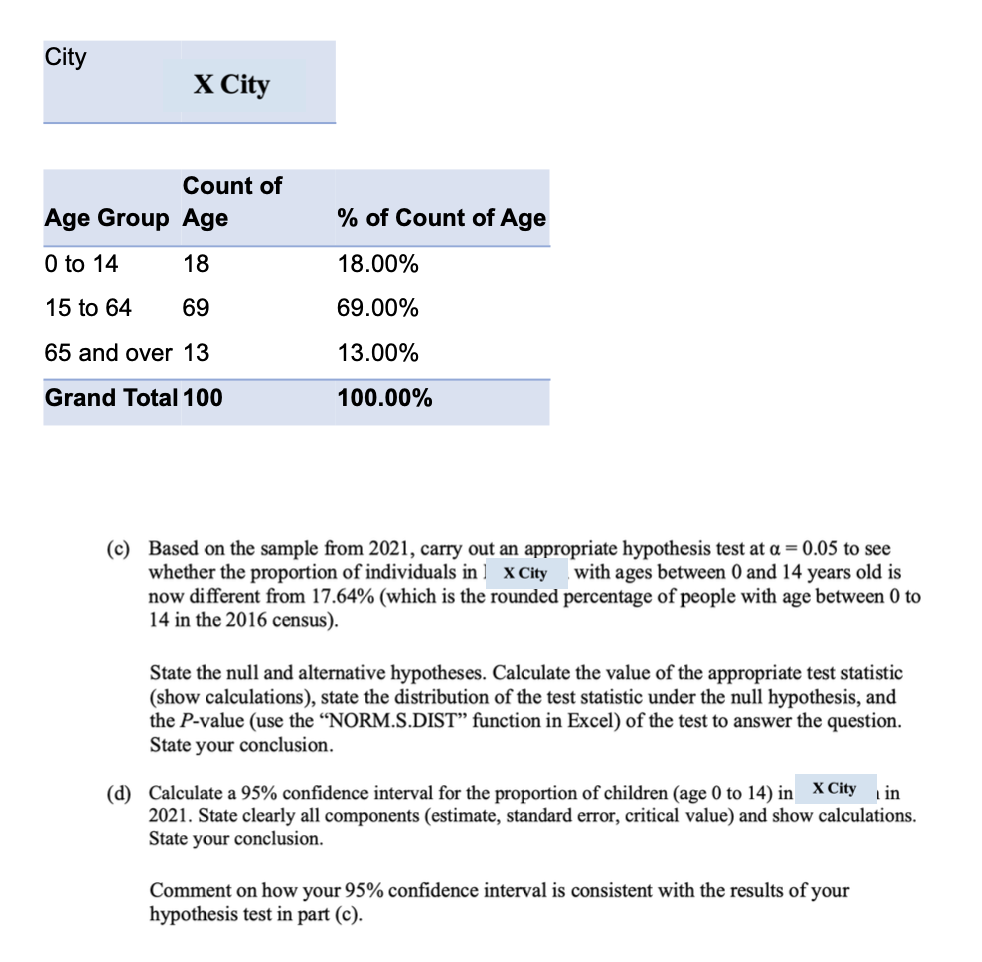# Question ​​​​​​​ (c) Based on the sample from 2021, carry out an appropriate hypothesis test at $$\alpha=0.05$$ to see whether the proportion of individuals in ] $$\mathbf{X}$$ City with ages between 0 and 14 years old is now different from $$17.64 \%$$ (which is the rounded percentage of people with age between 0 to 14 in the 2016 census). State the null and alternative hypotheses. Calculate the value of the appropriate test statistic (show calculations), state the distribution of the test statistic under the null hypothesis, and the $$P$$-value (use the "NORM.S.DIST" function in Excel) of the test to answer the question. State your conclusion. (d) Calculate a $$95 \%$$ confidence interval for the proportion of children (age 0 to 14) in in 2021. State clearly all components (estimate, standard error, critical value) and show calculations. State your conclusion. Comment on how your $$95 \%$$ confidence interval is consistent with the results of your hypothesis test in part (c).JRV50B The Asker · Probability and Statistics​​​​​​​

Transcribed Image Text: (c) Based on the sample from 2021, carry out an appropriate hypothesis test at $$\alpha=0.05$$ to see whether the proportion of individuals in ] $$\mathbf{X}$$ City with ages between 0 and 14 years old is now different from $$17.64 \%$$ (which is the rounded percentage of people with age between 0 to 14 in the 2016 census). State the null and alternative hypotheses. Calculate the value of the appropriate test statistic (show calculations), state the distribution of the test statistic under the null hypothesis, and the $$P$$-value (use the "NORM.S.DIST" function in Excel) of the test to answer the question. State your conclusion. (d) Calculate a $$95 \%$$ confidence interval for the proportion of children (age 0 to 14) in in 2021. State clearly all components (estimate, standard error, critical value) and show calculations. State your conclusion. Comment on how your $$95 \%$$ confidence interval is consistent with the results of your hypothesis test in part (c).
More
Transcribed Image Text: (c) Based on the sample from 2021, carry out an appropriate hypothesis test at $$\alpha=0.05$$ to see whether the proportion of individuals in ] $$\mathbf{X}$$ City with ages between 0 and 14 years old is now different from $$17.64 \%$$ (which is the rounded percentage of people with age between 0 to 14 in the 2016 census). State the null and alternative hypotheses. Calculate the value of the appropriate test statistic (show calculations), state the distribution of the test statistic under the null hypothesis, and the $$P$$-value (use the "NORM.S.DIST" function in Excel) of the test to answer the question. State your conclusion. (d) Calculate a $$95 \%$$ confidence interval for the proportion of children (age 0 to 14) in in 2021. State clearly all components (estimate, standard error, critical value) and show calculations. State your conclusion. Comment on how your $$95 \%$$ confidence interval is consistent with the results of your hypothesis test in part (c).&#12304;General guidance&#12305;The answer provided below has been developed in a clear step by step manner.Step1/4Let the sample proportion of 0 to 14 years old be $$\mathrm{\hat{{p}}={0.18}}$$sample size = n = 100.Let the true proportion of 0 to 14 years old be $$\mathrm{{p}={0.1764}}$$significance level $$\mathrm{\alpha={0.05}}$$Hypotheses:$$\mathrm{{H}_{{0}}:{p}={0.1764}}$$$$\mathrm{{H}_{{a}}:{p}\ne{0.1764}}$$This is a two-tail test.Explanation:Please refer to solution in this step.Step2/4Test statistic:$$\mathrm{{z}=\frac{{\hat{{p}}-{p}}}{\sqrt{{\frac{{{p}\times{\left({1}-{p}\right)}}}{{n}}}}}}$$where Z follows the standard normal distribution.$$\mathrm{{z}=\frac{{{0.18}-{0.1764}}}{\sqrt{{\frac{{{0.1764}\times{\left({1}-{0.1764}\right)}}}{{100}}}}}}$$$$\mathrm{{z}=\frac{{{0.0036}}}{\sqrt{{{0.1764}\times{\left({0.8236}\right)}}}}\times{10}}$$$$\mathrm{{z}={0.094}}$$The test statistic is 0.094The p-value:$$\mathrm{{p}-{v}{a}{l}{u}{e}={2}\times{P}{\left({Z}>{0.094}\right)}}$$From excel; The p-value is 0.925109Explanationexcel formula for p-value is:=2*(1-NORM.S.DIST(0.094,TRUE))Explanation:Please refer to solution in this step.Step3/4Decision Rule:Reject the null hypothesis $$\mathrm{{H}_{{0}}}$$ if the p-value is less than the significance level $$\mathrm{\alpha}$$.Do not reject the null hypothesis $$\mathrm{{H}_{{0}}}$$ if the p-value is greater than $$\mathrm{\alpha}$$.Conclusion: Since the p-value is greater than $$\mathrm{\alpha}$$, hence Do not reject the null hypothesis \( \mathrm{{ ... See the full answer